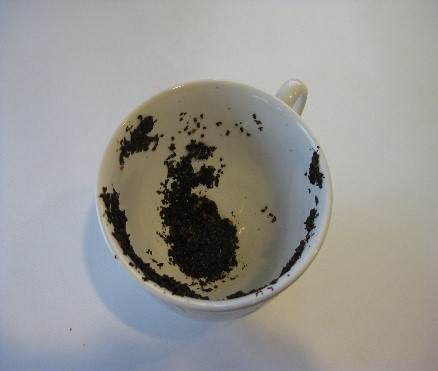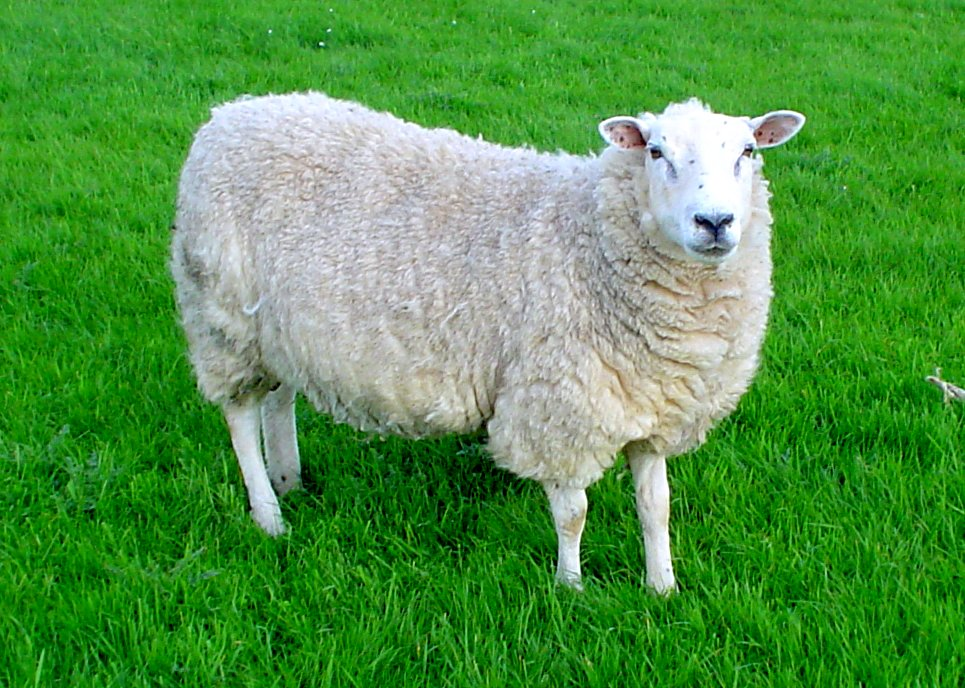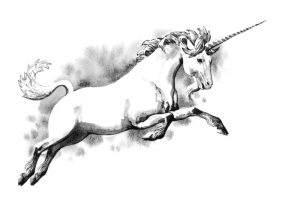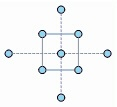How deeply has statistical content from Minitab blog posts (or other sources) seeped into your brain tissue? Rather than submit a biopsy specimen from your temporal lobe for analysis, take this short quiz to find out. Each question may have more than one correct answer. Good luck!

1.Which of the following are famous figure skating pairs, and which are methods for testing whether your data follow a normal distribution?

a. Belousova-Protopopov
b. Anderson-Darling
c. Kolmogorov-Smirnov
d. Shen-Zhao
e. Shapiro-Wilk
f. Salé-Pelletier
g. Ryan-Joiner

 Figure skaters are a, d, and f. Methods for testing normality are b, c, e, and g. To learn about the different methods for testing normality in Minitab, click here.

2.A t-value is so-named because...

a. Its value lies midway between the standard deviation(s) and the u-value coefficient (u).
b. It was first calculated in Fisher’s famous “Lady Tasting Tea” experiment.
c. It comes from a t-distribution.
d. It’s the first letter of the last name of the statistician who first defined it.
e. It was originally estimated by reading tea leaves.

 The correct answer is c. To find out what the t-value means, read this post.

3.How do you pronounce µ, the mean of the population, in English?

a. The way a cow sounds
b. The way a kitten sounds
c. The way a chicken sounds
d. The way a sheep sounds
e. The way a bullfrog sounds

 The correct answer is b. For the English pronunciation of µ and, more importantly, to understand how the population mean differs from the sample mean, read this post.4. What does it mean when we say a statistical test is “robust” to the assumption of normality?

a. The test strongly depends on having data that follow normal distribution.
b. The test can perform well even when the data do not strictly follow a normal distribution.
c. The test cannot be used with data that follow a normal distribution.
d. The test will never produce normal results.

 The correct answer is b. To find out which commonly used statistical tests are robust to the assumption of normality, see this post.

5.A Multi-Vari chart is used to...

a. Study patterns of variation from many possible causes.
b. Display positional or cyclical variations in processes.
c. Study variations within a subgroup, and between subgroups.
d. Obtain an overall view of the factor effects.
e. All of the above.
f. Ha! There’s no such thing as a “Multi-Vari chart!”

 The correct answer is e (or, equivalently, a, b, c, and d). To learn how you can use a Multi-Vari chart, see this post.

6.How can you identify a discrete distribution?

a. Determine whether the probabilities of all outcomes sum to 1.
b. Perform the Kelly-Banga Discreteness Test.
c. Assess the kurtosis value for the distribution.
d. You can’t—that’s why it’s discrete.

 The correct answer is a. To learn how to identify and use discrete distributions, see this post. For a general description of different data types, click here. If you incorrectly answered c, see this post.

7.Which of these events can be modeled by a Poisson process?

a. Getting pooped on by a bird
b. Dying from a horse kick while serving in the Prussian army
c. Tracking the location of an escaped zombie
d. Blinks of a human eye over 24-hour period
e. None of the above.

 The correct answer is a, b, and c. To understand how the Poisson process is used to model rare events, see the the following posts on Poisson and bird pooping, Poisson and escaped zombies, and Poisson and horse kicks.

8.Why should you examine a Residuals vs. Order Plot when you perform a regression analysis?

a. To identify non-random error, such as a time effect.
b. To verify that the order of the residuals matches the order of data in the worksheet.
c. Because a grumpy, finicky statistician said you have to.
d. To verify that the residuals have constant variance.

 The correct answer is a. For examples of how to interpret the Residuals vs Order plot in regression, see the following posts on snakes and alcohol, independence of the residuals, and residuals in DOE.

9. The Central Limit Theorem says that...

a. If you take a large number of independent, random samples from a population, the distribution of the samples approaches a normal distribution.
b. If you take a large number of independent, random samples from a population, the sample means will fall between well-defined confidence limits.
c. If you take a large number of independent, random samples from a population, the distribution of the sample means approaches a normal distribution.
d. If you take a large number of independent, random samples from a population, you must put them back immediately.

 The correct answer is c, although it is frequently misinterpreted as a. To better understand the central limit theorem, see this brief, introductory post on how it works, or this post that explains it with bunnies and dragons.10. You notice an extreme outlier in your data. What do you do?

a. Scream. Then try to hit it with a broom.
b. Highlight the row in the worksheet and press [Delete]
c. Multiply the outlier by e-1
d. Try to figure out what’s going on
e. Change the value to the sample mean
f.  Nothing. You’ve got bigger problems in life.

 The correct answer is d. Unfortunately, a, b, and f are common responses in practice. To see how to use brushing in Minitab graphs to investigate outliers, see this post. To see how to handle extreme outliers in a capability analysis, click here. To read about when it is and isn't appropriate to delete data values, see this post. To see what it feels like, statistically and personally, to be an outlier, click here.

11.Which of the following are true statements about the Box-Cox transformation?

a. The Box-Cox transformation can be used with regression analysis.
b. You can only use the Box-Cox transformation with positive data.
c. The Box-Cox transformation is not as powerful as the Johnson transformation.
d. The Box-Cox transformation transforms data into 3-dimensional cube space.

 a, b, and c are true statements. To see how the Box-Cox uses a logarithmic function to transform non-normal data, see this post. For an example of how to use the Box-Cox transformation when performing a regression analysis, see this post. For a comparison of the Box-Cox and Johnson transformations, see this post.

12.When would you use a paired t-test instead of a 2-sample t-test?

a. When you don’t get significant results using a 2-sample t test.
b. When you have dependent pairs of observations.
c. When you want to compare data in adjacent columns of the worksheet.
d. When you want to analyze the courtship behavior of exotic animals.

 The correct answer is b. For an explanation of the difference between a paired t test and a 2-sample t-test, click here.

13. Which of these are common pitfalls to avoid when interpreting regression results?a. Extrapolating predictions beyond the range of values in the sample data.
b. Confusing correlation with causation.
c. Using uncooked spaghetti to model linear trends.
d. Adding too much jitter to points on the scatterplot.
e. Assuming the R-squared value must always be high.
f. Treating the residuals as model errors.
g. Holding the graph upside-down.

 The correct answers are a, b, and e. To see an amusing example of extrapolating beyond the range of sample data values, click here. To understand why correlation doesn't imply causation, see this post. For another example, using NFL data, click here, and for yet another, using NBA data, click here. To understand what R-squared is, see this post. To learn why a high R-squared is not always good, and a low R-squared is not always bad, see this post.

14. Which of the following are terms associated with DOE (design of experiment), and which are terms associated with a BUCK?a. Center point
b. Crown tine
c. Main effect
d. Corner point
e. Pedicle
f.  Split plot
g. Block
h. Burr
i. Main beam
j. Run

 The design of experiment (DOE) terms are a, c, d, f, g, and j. The parts of a buck's antlers are b, e, and h. The Minitab blog contains many great posts on DOE, including several step-by-step examples that provide a clear, easy-to-understand synopsis of the process to follow when you create and analyze a designed experiment in Minitab. Click here to see a complete compilation of these DOE posts.

15. Which of these are frequently cited as common statistical errors?

a. Assuming that a small amount of random error is OK.
b. Assuming that you've proven the null hypothesis when the p-value is greater than 0.05.
c. Assuming that correlation implies causation.
d. Assuming that statistical significance implies practical significance.
e. Assuming that inferential statistics is a method of estimation.
f. Assuming that statisticians are always right.

 The correct answers are b, c, and d. To see common statistical mistakes you should avoid click here. And here.

## Looking for more information? Try the online Minitab Topic LibraryFor more information on the concepts covered in this quiz—as well as many other statistical concepts—check out the Minitab Topic Library.

On the Topic Library Overview page, click Menu to access topic of your choice.
For example, for more information on interpreting residual plots in regression analysis, click Modeling Statistics > Regression and correlation > Residuals and residual plots.